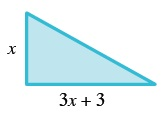### Home > A2C > Chapter 1 > Lesson 1.2.4 > Problem1-116

1-116.

The longer leg of a right triangle is three inches more than three times the length of the shorter leg. The area of the triangle is $84$ square inches. Find the perimeter of the triangle.

$A=\frac{1}{2}x(3x+3)$Substitute the value of the area, reorganize, and solve the resulting quadratic equation. Can you use both solutions?

Find the length of the hypotenuse by using the Pythagorean Theorem.

The perimeter is the length of the border of a shape.

$s=7$ inches
$I=24$ inches
hyp $=25$ inches
perimeter $=56$ inches